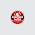Physics: Fundamental, derived quantity, Units, and Dimensions.

Fundamental Vs Derived quantity

Fundamental quantities

These are independent quantities with units. The dimension of a physical quantity indicates how it is made up in terms of S.I base quantity.

In other words, the physical quantities is said to have been expressed in terms of fundamental units [Length (L), Mass (M), Time (T), kelvin (k), mole etc].

Quantity Dimension

length         L
Mass          M
Time           T
Kelvin        K
Mole          m

Derived quantities:

These are obtained by some simple combinations of the fundamental quantities and units.

They are thus, dependent on the fundamental quantities and units. Example of the derived quantities, their derivation and their units are summarised in the table below:

 Derived quantity Derived unit Dimension Area  (length x breadth) m^2 L^2 Volume(length x breadth x height) m^3 L^3 Density (Mass/volume) kg/m^3 M/L/L Velocity (Displacement/time) m/s L/T Acceleration (Velocity/time) m/s/s L/T/T force (Mass x acceleration Newton (N) ML/T/T Energy & work (force x distance). joules ML^2/T/T power (work/time) joules/s ML^2/T/T/T Momentum (Mass x velocity) kg m/s ML/T Pressure  (force/area) N/m^2 ? Frequency (no of oscillation/time) 1/s 1/T

Watch video

Example

What is the dimension of EMF?

EMF(Electromotive Force) is a derived quantity. It is work done by the chemical force to move unit positive charge from negative terminal to positive terminal of the battery.
EMF = Workdone / charge
Work=Force * displacement
=MLT^-2 * L =ML^2T^-2
Charge=Current * time
=IT
Now,
Dimensional formula of
EMF=Work/charge
=ML^2T^-2 / IT
=ML^2T^-3I^-1

Exercise:

What is the dimension of pressure?

Solution
Watch how to derive the dimension of Pressure.

Conclusion

A  derived quantity is derived from fundamental quantity, simple and short. I hcomments article was helpful. If you have any questions or comments, please don't hesitate to write it in the comments section. All comments are appreciated.

Previous Post
Next Post

1 comment:

1.What is the dimension
of pressure?

ML^-1 T^-2